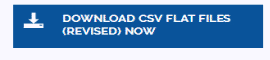# Review of Probability and Distributions

This week we talk through how we can measure and describe uncertainty.  When a person says to his partner “I am not sure where this relationship is going?” what does that mean precisely?   Can we assign numbers to our uncertainty about the future, even uncertainty about love.  We are all familiar with probability as a frequency of an event but not as a strength of our belief in the likelihood of the event. This week we look at probability and its calculus as a method of organizing opinions.  The neat part of this week is the procedure for estimating the probability of rare events.  In healthcare, adverse events such as wrong side surgery are rare. This week, we learn how to accurately measure rate of occurrence of rare events.  When it comes to acting under uncertainty, one needs to estimate conditional probability, where the probability of outcome is calculated under different actions. Conditional probabilities are calculated by shrinking the universe of possibilities. Once conditional probabilities are calculated, expectation is used to recommend action under uncertain situations. Enough about what is coming up, let us proceed.

This section of the course is a review of material you have had in an introductory course on statistics

• Introduction to probability (use instructor's last name as password) Read►

# Presentations

1. Deﬁning probability  Slides► YouTube►
2. Probability calculus  Slides► YouTube►
3. Probability distributions & expectations  Slides► Video► YouTube►
4. Mathematical concept of expectation Slides► YouTube►
5. Expected variability in random variables  Slides►  Video►  YouTube►
6. Descriptive statistics  Slides► Video►
7. Measures of variability Video►  Slides►  YouTube►
8. SPSS Tutorial 1►  Tutorial 2►
9. Population and sampling You Tube►
10. Observational studies   Slides► Video► YouTube►
11. Numerical data  YouTube► Excel►
12. Data basics Slides► Video► YouTube►
13. Data matrices and types of variables Slides► Video►  YouTube►
14. Variance and standard deviation Slides►  Video►  YouTube►
15. Box plots, quartiles, and the median How to►
16. Histograms and shape  How to►  How to►  YouTube►

# Assignments

Instruction for Submission of Assignments: Assignments should be submitted directly on Blackboard.  In rare situations assignments can be sent directly by email to the instructor. Submission should follow these rules:

1.  Submit only one document, usually an Excel file.  All questions should be answered in different sheets.  Each sheet should be labeled with the question number.
2. All Excel cells, except the cells containing the data, must have formulas.  Do not paste the value into the cell, it must be calculated using a formula.  Even simple steps, such as adding two numbers, should be done using formulas.
3. Make sure that any control charts follow the visual rules below:  (1) Control limits must be in red and without markers, (2) Observed lines must have markers, (3) X and Y axis must be labeled, and (4) Charts must be linked to the data.
4. Copy and paste SQL or R code into Excel sheet.  Plot data in Excel.

Question 1: In this problem we ask you to calculate a case mix index for a hospital from classification of its patients into Diagnostic Related Groupings (DRGs).  In Health Administration programs case mix issues arises in multiple courses where severity of patients receiving care in different hospitals are discussed.  The case mix index allows the comparison of two hospitals. It is generally calculated as a weighted length of stay across all DRGs see in the hospital. The concept of weighted average was discussed in this section.  In a case mix index, the weights are the probability of observing patients in a particular DRG category.  Each DRG category is assumed to be mutually exclusive and exhaustive.  The number of patients who are admitted for different DRGs are indicated in the attached data file.  From these numbers you calculate the probability of each DRG.  By multiplying the probability of the DRG by length of stay you get the contribution of each DRG.  The case mix index is the sum of the product of probability of each DRG and length of stay within each DRG.  The higher the case mix index, the larger the expected length of stay at the hospital. Which hospital has a higher case mix index?  Data► Answer► Anto's Teach One►